Students can download Maths Chapter 6 Information Processing Ex 6.2 Questions and Answers, Notes, Samacheer Kalvi 6th Maths Guide Pdf helps you to revise the complete Tamilnadu State Board New Syllabus, helps students complete homework assignments and to score high marks in board exams.

## Tamilnadu Samacheer Kalvi 6th Maths Solutions Term 1 Chapter 6 Information Processing Ex 6.2

Question 1.
In the following magic triangle, arrange the numbers from 1 to 6, so that you get the same sum on all its sides.Solution: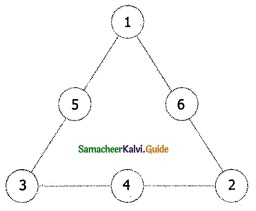Question 2.
Using the numbers from 1 to 9
(i) Can you form a magic triangle?
(ii) How many magic triangles can be formed?
(iii) What are the sums of the sides of the magic triangle?Solution:
(i) Yes
(ii) 5
(iii) 17, 19, 20, 21, 23Question 3.
Arrange the odd numbers from 1 to 17 without repetition to get a sum of 30 on each side of the magic triangle.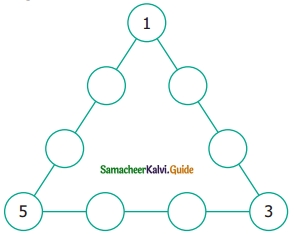Solution: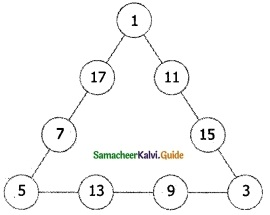Question 4.
Put the numbers 1, 2, 3, 4, 5, 6 & 7 in the circles so that each straight line of three numbers add up to the same total.Solution: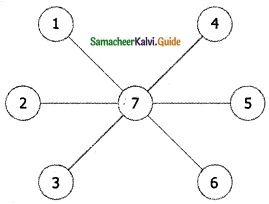Question 5.
Place the number 1 to 12 in the 12 circles so that the sum of the numbers in each of the six lines of the star is 26. Use each number from 1 to 12 exactly once. Find more possible ways?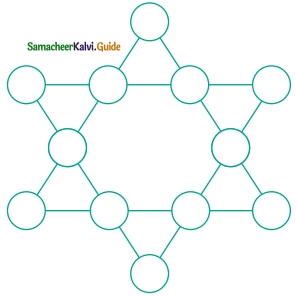Solution: